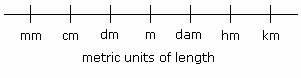## Definition Of Metric System

Metric System is a base ten system of measurement, where each unit is defined in terms of multiples of 10.The above figure shows the consecutive metric units of length. This is used in conversion of units of length easily.
10 millimeter (mm) = 1 centimeter (cm)
10 centimeter cm = 1 decimeter (dm)
10 decimeter (dm) = 1 meters (m)
1 dekameter (dam) = 10 meters (m)
hectometer (hm) = 10 dekameter (dam)
kilometer (km) = 10 hectometer (hm)

### Examples of Metric System

As the Metric System uses base ten system of measurement, each unit can easily be converted into other and vice-versa.
900 cm can be converted to meter by using relationship 1 m =100 cm.
So, 900 cm = 9 × 100 cm = 9 m.

A. 4940 m
B. 49.4 m
C. 49400 m
D. 494 m